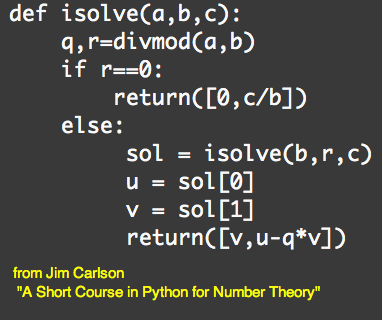Solving Diophantine Equations
17feb11

\begin{document}
\maketitle\section{Introduction}
In this lesson we study Jim Carlson's algorithm for solving a Diophantine
equation using Python and recursion. It is taken from the web document
Jim Carlson
"A Short Course in Python for Number Theory"  p11,12.

\section{The Solution}

\subsection{Some notation}
\textit{ Parentheses, brackets and braces.} \\
Parentheses in mathematics have many meanings, depending
on the context. But computers are not able to handle context. So
we must distinguish their use for grouping arithmetic expressions.
We can also leave them for writing functions because the name of
the function preceeding their use establishes the functional context.
But for ordered pairs of numbers, we'll switch to square brackets.
Recall that is LaTeX braces take on very special grouping role. In
some computer languages, braces take on a similar role.

\textit{ The equals sign.} \\
In mathematics the equals sign always means an equation, which may
or may not be true. But it must be capable of being true or false.
We also use the equal sign when we substitute a single letter for
a more complicated expression.  Sometimes, we use := for this purpose.
But it is always correct to exchange right and left sides of an equation.
In computer languages the equals sign means quite something different,
and you may never exchange the sides here. The equal sign in Python
as in other computer languates means that you assgn the value of the
expression on the RHS to the object named on the LHS.

\subsection{The algorithm}
We want to solve
\begin{eqnarray*}  ax+by&=&c \mbox{    .......... (1) } \\ \end{eqnarray*}
Assuming that $gcd(a,b)|c$ else there is no solution. \\
Do a long-division step
\begin{eqnarray*}  a&=&bq+r  \mbox{    .......... (2) } \\ \end{eqnarray*}
If $r=0$ then there is a trivial solution of (1) with $x=0, y=c/b$.

Else, we get an equivalent equation by substituting and rewriting.
\begin{eqnarray*}
(bq+r)x+by&=&c  \mbox{    .......... (3) } \\
b(qx+y)+r(x)&=&c  \mbox{    .......... (4) } \\
b(u)+r(v)&=&c  \mbox{    .......... (5) } \\
\end{eqnarray*}
where $[u,v]=[qx+y,x]$ is a just a renaming, which is invertible.
\begin{eqnarray*}  [x,y]&=&[v,u-qv]  \mbox{    .......... (6) } \\ \end{eqnarray*}

That is, if $[u,v]$ is the solution to $bu + rv=c$ then (6) yield
the solution to $ax+by=c$.

\subsection{The code}
Jim Carlson's function \texttt{ isolve(a,b,c)} implements this algorithm recursively.
The variable \texttt{sol} denotes the output of \texttt{ isolve } applied after
a long division step. The output is an ordered pair, the first element of which
is \texttt{ sol } and the second element is \texttt{ sol}. In mathematics,
we would not use a multiletter word for a vector $s=[s_0,s_1]$, and we would use
\end{document}